# Geometric Inversion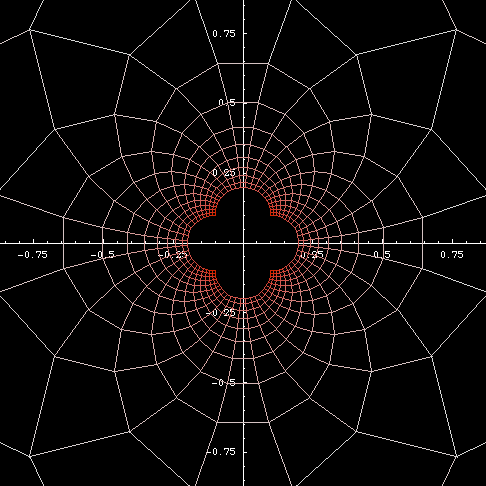Inversion of a rectangular grid. (only the grid points are inverted, then they are connected by lines after the inversion).

## History

Geometrical inversion seems to be due to Jakob Steiner (“the greatest geometer since Apollonius”) who indicated a knowledge of the subject in 1824. He was closely followed by Adolphe Quetelet (1825) who gave some examples. Apparently independently discovered by Giusto Bellavitis in 1836, by Stubbs and Ingram in 1842-3, and by Lord Kelvin in 1845. The latter employed the idea with conspicuous success in his electrical researches. (Robert Yates: Curves and Their Properties.)

## Description

### Geometric Inversion

Inversion in geometry is a transformation. Let P be a given point. Let c be a circle centered on O and radius r. The inverse of P with respect to c is a point Q on the line[O,P] such that distance[O,P] * distance[O,Q] == r^2.

From this definition, two properties can be seen easily: (1) Q is a inverse of P if and only if P is a inverse of Q. (2) Points inside the circle are mapped to the outside and vice versa. Points on the circle are fixed points (i.e. the inversion of any point on the inversion circle is itself). As P moves futher from O, its image Q moves closer to O. From this observation, we may then define the inverse of a point on the center of the inversion circle to be a point at infinity, and vice versa. With such a definition, we have obtained a transformation on a plane that has a point at infinity. This concept is important in geometry, and is used in Spherical Projection. It shows that such a plane is topologically equivalent to a sphere. Also, inversion can be thought of as a generalization of reflection. A normal reflection is then a inversion with the inversion circle radius infinitly large.

### Inversion Of A Curve

The inversion of a curve is the inversion of all points on the curve. It can be thought of as a way to derive a new curve based on a given curve and a circle. If curve A is the inverse of curve B, then curve B is also the inverse of curve A with respect to the same circle. The center of the inversion circle is called the pole. One property easily seen from the definition is that the radius of inversion circle effects the scale of the inversion curve, but does not effect its shape. Curves that inverts into themselves are called anallagmatic curves. Circles, lines, and Cassinian ovals are anallagmatic curves. Asymptotes to a curve C invert into a curve that is tangent C's inverse.

Step by step description:

1. Given a curve C, and a circle M centered at O with radius r.
2. Draw a line passing O and any point P on the curve.
3. Mark a point Q on this line such that distance[O,P] * distance[O,Q] == r^2.
4. Repeat this for other points P on the curve.
5. The locus of Q is the inverse of curve C with respect to circle M.

## Formula

### Point in Rectangular Coordinate

The inversion of a point {x,y} with respect to a circle centered at {a,b} and radius r is:

```{x-a,y-b}*r^2/((x-a)^2+(y-b)^2) + {a,b}
```

The derivation can be easily done by thinking in vectors. Suppose we have a vector P with coordinates {x,y}. We want to find a vector Q such that length[P]*length[Q]==r^2. Q would be the inverse of P with respect to a circle of radius r at origin. Now, the length of P is Sqrt[x^2+y^2]. We have length[Q]==r^2/Sqrt[x^2+y^2]. The vector Q is a vector in the direction of P with length r^2/Sqrt[x^2+y^2]. We multiply the unit vector in the direction of P with the desired length: (P/length[P])*(r^2/Sqrt[x^2+y^2]), which simplifies to {x,y}*r^2/(x^2+y^2). This would be the formula for inversion of a point {x,y} with respect to a circle centered on origin with radius r.

Now, if the pole O is not at the origin, we can derive the formula by first translating O and P so that O is at the origin, then do the inversion, then translate the inverted point in reverse direction. In formulas, suppose the component of O is {a,b}, the coordinate of P' is then {x-a,y-b} and O is {0,0}. Applying the inversion formula {x,y}*r^2/(x^2+y^2), we have {x-a,y-b}*r^2/((x-a)^2+(y-b)^2). Translate it back, we have: {x-a,y-b}*r^2/((x-a)^2+(y-b)^2) + {a,b}.

### Polar Coordinate

Give a curve with polar formula r==f[θ], its inverse curve with respect to a circle centered on the pole with radius k is r==k^2/f[θ].

Give a curve with parametric formula in polar coordinate: {f[θ],θ}, its inverse curve with respect to a circle centered on the pole with radius k is {k^2/f[θ],θ}.

## Properties

### Inversion of Circle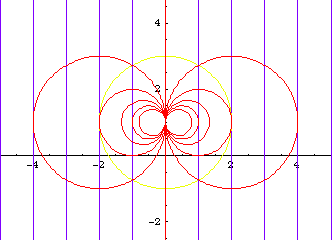Inversion of parallel lines and co-linear circles.

The inversion of a circle or line is a circle or line. Let O denote the center of inversion. We have:

Curve ACurve B
line through Oline through O
line not through Ocircle through O
circle through Oline not through O
circle not through Ocircle not through O

Proof: Statement Ⓐ is trivial. To prove Ⓑ, let A be a point on the line L so that OA and L are perpendicular. Let P be any point on L other than A. Let A' and P' be the inverse of A and P. By the definition of inversion, we have OA'*OA == OP'*OP == r^2. By algebra we have OA'/OP' == OP/OA. This means triangle[O,P',A'] and triangle[O,A,P] are similar because the ratio of their sides are equal, therefore angle OP'A' is a right angle. From the Converse of Thales's Theorem , it follows that P' lies on a circle with diameter OA'. This ends the proof of statement Ⓑ. Proof of statement Ⓒ follows automatically because inversion of points are mutual.

Proof of Ⓓ: Let the intersection of the circle and a line thourgh O be P and Q. Let P', Q' be their images. Let M be the center of the given circle. We have OP*OP' == OQ*OQ' == r^2 by the definition of inverse. Also, OP*OQ == OM^2-r^2 from a elementary theorem on circle. Divide the first equation by the second we get OP'/OQ == OQ'/OP == r^2/(OM^2-r^2), which is a constant. Through Q' we draw a line parallel to PM. Let the intersection of the line and OM be N. We have now triangles OQ'N and OPM. Since these triangles has two sides parallel, they must be similar, thus OQ'/OP == Q'N/PM == NO/MO. Solve for NO we get NO == MO*OQ'/OP == MO*r^2/(OM^2-r^2). Solve for Q'N we have Q'N == PM*OQ'/OP == r*r^2/(OM^2-r^2). Both of which is a constant. This means for any point P on the circle, Q'N and NO are constant. Similarly, for any point Q on the circle, P'N and NO are constant. Thus P' and Q' must be points on the same circle. End of proof.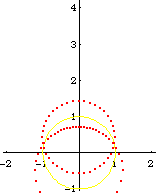Inversion of a moving circle. The yellow circle is inversion circle. Inversion of A Circle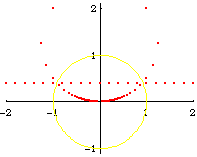Inversion of a moving line. The yellow circle is inversion circle. Inversion of A Line

From the formula of a inversion of a point, and the theorem that circle invert to circles, we can easily derive the formula of a Circle[{c1, c2}, s] inverted wrt Circle[{o1, o2}, r], is:

```Circle[({c1, c2} - {o1, o2})/oc*(oc r^2/(oc^2 - s^2)) + {o1, o2},
Abs[r^2 s/(oc^2 - s^2)]]
```

where oc is the distance from {c1,c2} to {o1,o2}

### Invariance of Angles

Angles are preserved under inversion with reversed sense. That is, if two curves intersect with angle α, their inverse will also intersect with angle α but in a reversed direction of sweeping.

Inversion Angle InvarianceInversion of a finite rectangular grid. Note that the flower-shaped hole in the center is originally the edge boundary of the grid. Note that line segments invert to incomplete circles. Note that circles intersect in right angles. The inversion of a rectangular grid becomes essentially circles with centers lying on the x-axis and y-axis, and all circles sharing a common point at the origin.

Peaucellier Linkage is a mechanical contrivance for tracing the inverse of a curve. It is named after Charles-Nicolas Peaucellier (1832 to 1913) who discovered it in 1864. The significance of this simple linkage is that it solved the problem of converting a circular motion to a linear motion by a linkage that was believed to be impossible at the time.

The linkage consists of six arms of fixed length (green and light blue lines in figure above), and five joints (point O, P, Q, E, D). To generate a inverse of a given curve (For example, a line in blue), fix point O in the plane and move P along the curve. The trace of Q will be the inverse of C with respect to O and radius Sqrt[a^2-b^2], where a and b are the length of the arms. To convert circular motion to linear motion, we simply need to restrain the motion of Q on a fixed circle passing O.

Proof: We need to show such device with any degree of opening will have OP * OQ == r^2 for a constant r. Let x = ED/2, thus x represents the variable opening of the device. We have OP == Sqrt[a^2-x^2] + Sqrt[b^2-x^2], OQ == Sqrt[a^2-x^2] - Sqrt[b^2-x^2]. Then OP * OQ == a^2-b^2, after simplification. End of Proof.

Hart's Linkage is another mechanical contrivance for tracing the inverse of a curve. The linkage consists of four arms of fixed length (green and light blue lines in figure below), four joints (point A, B, C, D), and three positions (O, P, Q) fixed on the arms. The two lengths (AB==CD, BC==AD) of the four arms are arbitrary. The fixed positions O, P, Q on the arms are also arbitrary with the condition that O, P, Q must be collinear and parallel to AC. To trace a inverse of a given curve (For example, a circle in blue), fix point O in the plane and move P along the curve. The trace of Q (pink) will be the inverse with respect to O.

Proof: We want to show that OP * OQ == r^2 for some constant r, no matter what design or position of Hart's linkage. We note that points A, B are symmetric to C, D because AB==CD, BC==AD, thus AC, BD, OP are all parallel. Triangle AOP and ABD are simular, thus OP/BD == AO/AB. Similarly, triangle BOQ and BAC implies OQ/AC == BO/BA. Combine the last two equations we get OP*OQ == (AO)*(BO)*(BD)*(AC)/(AB)^2. Now we just need to show the quantity (BD)*(AC) is a constant. Let E, F be the parallel projection of point A, C on the line B, D. So we have right triangles AEB and CFD. Now BD*AC == BD*EF == (ED+EB)*(ED-EB) == ED^2 - EB^2. We know that ED^2 + AE^2 == AD^2 and EB^2 + AE^2 == AB^2. Combine the last three equations to get ED^2 - EB^2 == AD^2 - AB^2. This quantity is a constant regardless the positions of the linkage. End of Proof. We have derived the radius of inversion with Hart's linkage is r == Sqrt[ (AO)*(BO)*/(AB)^2*(AD^2 - AB^2)].

Note that if we constrain point P of Peaucellier or Hart's linkage to move around a circle that passes point O, we have a mechanism that converts circular motion to linear motion.

### Curve relations by inversion

Curve Center of Inversion Curve
line not on line; on circumference circle
circle not on circumference circle
conics focus; pole limacon of Pascal
ellipse, hyperbola center Oval?, lemniscate of Gerono
parabola focus; cusp cardioid
parabola vertex; cusp cissoid of Diocles
rectangular hyperbola center lemniscate of Bernoulli
rectangular hyperbola vertex; node right strophoid
hyperbola, e:=2/Sqrt vertex; node trisectrix of Maclaurin
trisectrix of Maclaurin focus; -- Tschirnhausen's cubic
right strophoid pole right strophoid
equiangular spiral pole equiangular spiral
Archimedean spiral pole Archimedean spiral
lituus pole parabolic spiral
sinusoidal spiral pole sinusoidal spiral
epispiral pole rose
cochleoid pole; -- quadratrix of Hippias

### Misc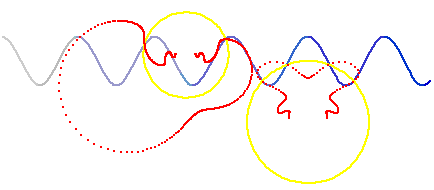Two inversions of a sin curve. Inversion of Sine Curve

## Related Web Sites

Robert Yates: Curves and Their Properties .